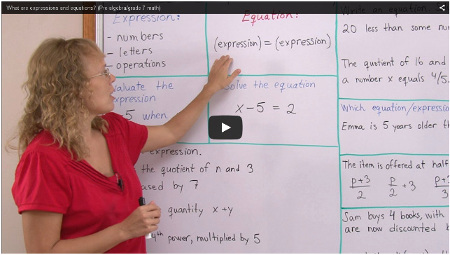^

# Free videos on expressions and ecuations (grades 6-7)

Find here free online math videos on algebraic expressions and equations for middle school. The videos match lessons in the Blue Series books Expressions & Equations and Linear Equations.

## Introduction to algebra

Expressions – What are expressions and how to evaluate them

Expressions – write expressions from word phrases

Writing and simplifying expressions 1: length and perimeter

Writing and simplifying expressions 2: area

The distributive property (simplified lesson, for 6th grade)

Factoring linear expressions

Equations (an introduction)

Inequalities

Using two variables – an introduction to equations with two variables

## The language of algebra

The topics below provide an foundation to principles used in algebra (and pre-algebra).The order of operations

What are expressions & equations?

Working with expressions & equations

The commutative & associative properties

The distributive property — for grade 7 or pre-algebra

## Growing patterns

I have not made video lessons for growing patterns, but you can use this Youtube video for starters.

The other two I list below give a very mechanical way of approaching these types of pattern problems, where you don't have to think so much — but the method explained works always, so it can be helpful, especially if such a problem is expected to be on a standardized test:

However, my goal in putting the growing pattern problems in Math Mammoth materials is NOT to teach students a mechanical way, but just give them some exposure to thinking of different ways to SEE the pattern, and then try to express it using symbols. So there is no need to learn the mechanical way.

## Linear equations

Two-step equations

Variable on both sides

Equations involving parentheses

Equations with fractions

How to use the formula for distance, rate (speed), and time to solve problems

Writing equations for word problems

Inequalities

Graphing equations

Introduction to slope

The above videos match lessons in the Blue Series books Expressions & Equations and Linear Equations.

Math Mammoth Expressions & Equations worktext

Math Mammoth Linear Equations worktext

WAIT!

Receive my monthly collection of math tips & resources directly in your inbox — and get a FREE Math Mammoth book!You can unsubscribe at any time.

### Math Mammoth Tour

Confused about the different options? Take a virtual email tour around Math Mammoth! You'll receive:

An initial email to download your GIFT of over 400 free worksheets and sample pages from my books. Six other "TOURSTOP" emails that explain the important things and commonly asked questions concerning Math Mammoth curriculum. (Find out the differences between all these different-colored series!)

This way, you'll have time to digest the information over one or two weeks, plus an opportunity to ask me personally about the curriculum.
A monthly collection of math teaching tips & Math Mammoth updates (unsubscribe any time)### "Mini" Math Teaching Course

This is a little "virtual" 2-week course, where you will receive emails on important topics on teaching math, including:

- How to help a student who is behind
- Troubles with word problems
- Teaching multiplication tables
- Why fractions are so difficult
- The value of mistakes
- Should you use timed tests
- And more!

A GIFT of over 400 free worksheets and sample pages from my books right in the very beginning.A monthly collection of math teaching tips & Math Mammoth updates (unsubscribe any time)
Enter your email to receive math teaching tips, resources, Math Mammoth news & sales, humor, and more! I tend to send out these tips about once monthly, near the beginning of the month, but occasionally you may hear from me twice per month (and sometimes less often).• A GIFT of over 400 free worksheets and sample pages from my books.